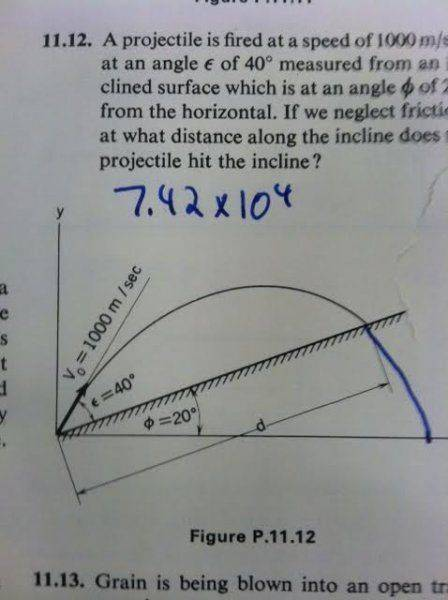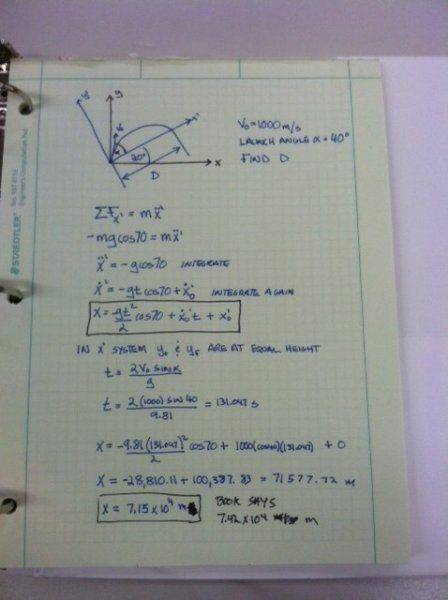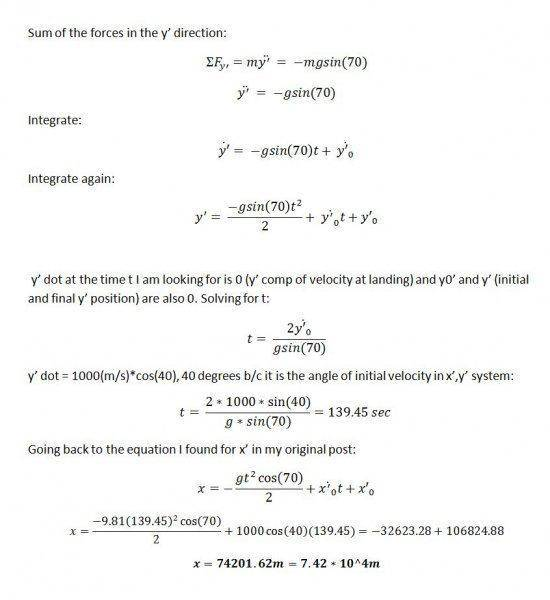# Projectile Motion With Unequal Launch And Landing Height

## Homework Statement

Below is screen shot of the problem. My friends book was ripped so some of the problem was cut off. Using the diagram I need to find d. The answer is supposed to be 7.42x10^4m. Below the problem is my attempt at the solution. Can someone tell me where I went wrong. As you can see from my solution my answer is slightly different. Thanks.## The Attempt at a Solution

If it is hard to tell from my scanned copy I use X' to denote the co-ordinate system that is rotated 20 degrees and I use dots above the x to denote derivatives. X double dot is acceleration and so forth.SteamKing
Staff Emeritus
Homework Helper
It looks like you are using 70° as the angle the initial velocity makes with the x-axis. Is this some kind of 'new math' I'm not familiar with?

The initial velocity makes an angle of 60 degrees with the x axis. That is clear from the diagram from the book.

In my attempt at the solution I drew the x',y' coordinate system rotated 20 degrees CCW from x,y. I did this so the launch height and the landing height would be the same. The 70 degrees comes from the component of acceleration from gravity that acts in the x' direction. If it was just x,y obviously gravity would be only in the -y direction but since I aligned my co-ordinate system with the 20 degree inclined plane shown in the problem I had to compensate for that. This could be where I went wrong and that is why I am asking.

Mark44
Mentor
The initial velocity makes an angle of 60 degrees with the x axis. That is clear from the diagram from the book.

In my attempt at the solution I drew the x',y' coordinate system rotated 20 degrees CCW from x,y. I did this so the launch height and the landing height would be the same. The 70 degrees comes from the component of acceleration from gravity that acts in the x' direction. If it was just x,y obviously gravity would be only in the -y direction but since I aligned my co-ordinate system with the 20 degree inclined plane shown in the problem I had to compensate for that. This could be where I went wrong and that is why I am asking.
IMO changing the coordinate system is not the right way to approach this, since gravity will still be acting straight down. Break up the velocity vector into its vertical and horizontal components and use right triangle trig to find the coordinates of the impact point on the slope.

•SteamKing
Ok I will try it that way. I thought it may have been easier to have x' aligned with the incline but that is where things probably went wrong. Thanks.

I did the problem in the standard x-y co-ordinate system and got the right answer that the book had.

After I finished I realized that I used the same time equation for both the flat and rotated co-ordinate system. Since that equation comes from the sum of the forces in y for whatever system you are working in that is where I went wrong in my first attempt. Below is the rest of the solution of the 20 deg. rotated co-ordinate system. With the correct equation for time they both give the same answer.

Looking back thou Mark44 is right it is simpler to stick with the standard x-y system.